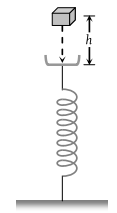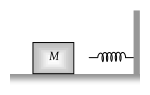If a man increase his speed by 2 m/s , his K.E. is doubled, the original speed of the man is

(1) $\left(1+2\sqrt{2}\right)\text{\hspace{0.17em}}m/s$

(2) 4 m/s

(3) $\left(2+2\sqrt{2}\right)\text{\hspace{0.17em}}m/s$

(4) $\left(2+\sqrt{2}\right)\text{\hspace{0.17em}}m/s$

Concept Questions :-

Concept of work
High Yielding Test Series + Question Bank - NEET 2020

Difficulty Level:

A block of mass m initially at rest is dropped from a height h on to a spring of force constant k. the maximum compression in the spring is x then [BCECE 2005](1) $mgh=\frac{1}{2}k{x}^{2}$

(2) $mg\left(h+x\right)=\frac{1}{2}k{x}^{2}$

(3) $mgh=\frac{1}{2}k{\left(x+h\right)}^{2}$

(4) $mg\left(h+x\right)=\frac{1}{2}k{\left(x+h\right)}^{2}$

Concept Questions :-

Elastic potential energy
High Yielding Test Series + Question Bank - NEET 2020

Difficulty Level:

A spherical ball of mass 20 kg is stationary at the top of a hill of height 100 m. It slides down a smooth surface to the ground, then climbs up another hill of height 30 m and finally slides down to a horizontal base at a height of 20 m above the ground. The velocity attained by the ball is

(1) 10 m/s

(2) $10\sqrt{30}$ m/s

(3) 40 m/s

(4) 20 m/s

Concept Questions :-

Gravitational Potential energy
High Yielding Test Series + Question Bank - NEET 2020

Difficulty Level:

The block of mass M moving on the frictionless horizontal surface collides with the spring of spring constant K and compresses it by length L. The maximum momentum of the block after collision is(1) Zero

(2) $\frac{M{L}^{2}}{K}$

(3) $\sqrt{MK}\text{\hspace{0.17em}}L$

(4) $\frac{K{L}^{2}}{2M}$

Concept Questions :-

Elastic potential energy
High Yielding Test Series + Question Bank - NEET 2020

Difficulty Level:

A body of mass m accelerates uniformly from rest to v1 in time t1. As a function of time t, the instantaneous power delivered to the body is

(1) $\frac{m{v}_{1}t}{{t}_{1}}$

(2) $\frac{m{v}_{1}^{2}t}{{t}_{1}}$

(3) $\frac{m{v}_{1}{t}^{2}}{{t}_{1}}$

(4) $\frac{m{v}_{1}^{2}t}{{t}_{1}^{2}}$

Concept Questions :-

Power
High Yielding Test Series + Question Bank - NEET 2020

Difficulty Level:

A weight lifter lifts 300 kg from the ground to a height of 2 meter in 3 second. The average power generated by him is

(1) 5880 watt

(2) 4410 watt

(3) 2205 watt

(4) 1960 watt

Concept Questions :-

Power
High Yielding Test Series + Question Bank - NEET 2020

Difficulty Level:

The average power required to lift a 100 kg mass through a height of 50 metres in approximately 50 seconds would be

(1) 50 J/s

(2) 5000 J/s

(3) 100 J/s

(4) 980 J/s

Concept Questions :-

Power
High Yielding Test Series + Question Bank - NEET 2020

Difficulty Level:

The power of a pump, which can pump 200kg of water to a height of 200m in 10sec is (g = 10 m/s2)

(1) 40 kW

(2) 80 kW

(3) 400 kW

(4) 960 kW

Concept Questions :-

Power
High Yielding Test Series + Question Bank - NEET 2020

Difficulty Level:

A 60 kg man runs up a staircase in 12 seconds while a 50 kg man runs up the same staircase in 11, seconds, the ratio of the rate of doing their work is

(1) 6 : 5

(2) 12 : 11

(3) 11 : 10

(4) 10 : 11

Concept Questions :-

Power
High Yielding Test Series + Question Bank - NEET 2020

Difficulty Level:

What average horsepower is developed by an 80 kg man while climbing in 10 s a flight of stairs that rises 6 m vertically

(1) 0.63 HP

(2) 1.26 HP

(3) 1.8 HP

(4) 2.1 HP

Concept Questions :-

Power